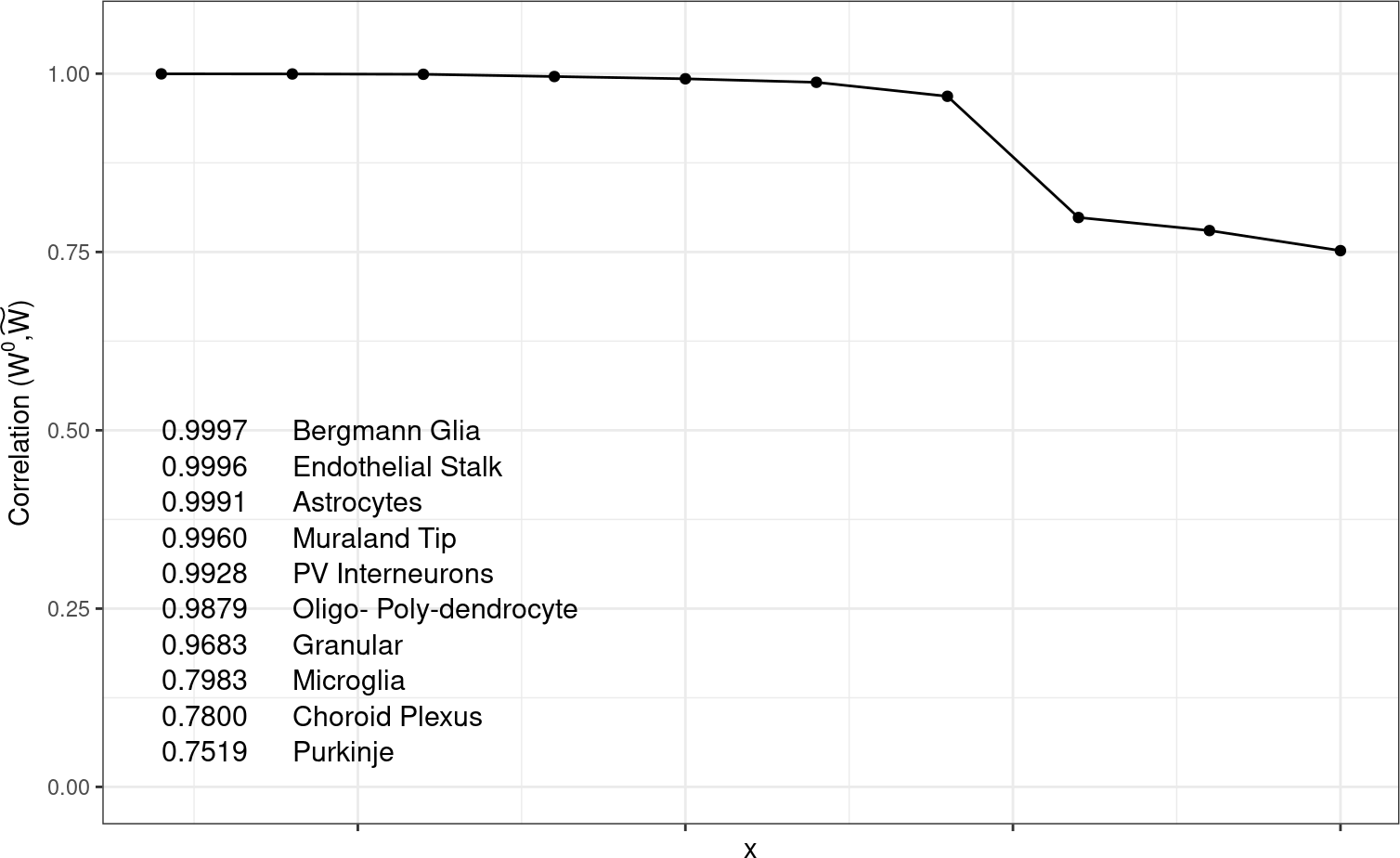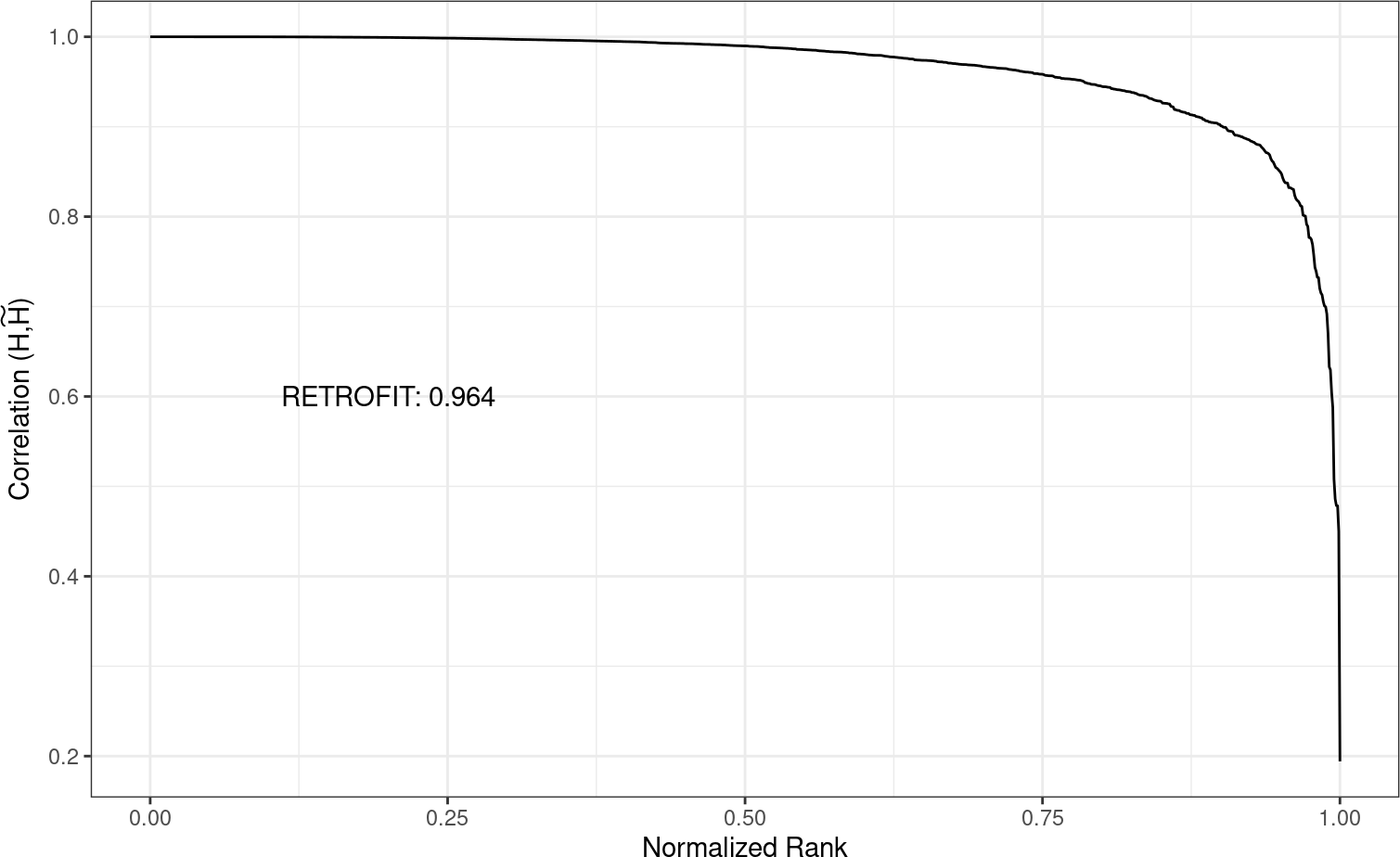# 1 Introduction

RETROFIT is a statistical method for reference-free deconvolution of spatial transcriptomics data to estimate cell type mixtures. In this Vignette, we will estimate cell type composition of a sample synthetic dataset. We will annotate cell types using an annotated single cell reference.

# 2 Package Installation

Install and load the package using the following steps:

if(!requireNamespace("BiocManager", quietly = TRUE))
install.packages("BiocManager")
BiocManager::install("retrofit")

The main functionalities of RETROFIT are covered in this tutorial.

# 3 Spatial Transcriptomics Data

First load the ST data, using the following command:

data("vignetteSimulationData")
x = vignetteSimulationData$n10m3_x The ST data matrix will consist of G = 500 genes and S = 1000 spots i.e., a matrix of order G x S. # 4 Deconvolution Initialize the following parameters for deconvolution: - iterations: Number of iterations (default = 4000) - L: Number of components required iterations = 10 L = 20 After initialization, run RETROFIT on the data (X) as follows: result = retrofit::decompose(x, L=L, iterations=iterations, verbose=TRUE) ##  "iteration: 1 0.098 Seconds" ##  "iteration: 2 0.092 Seconds" ##  "iteration: 3 0.097 Seconds" ##  "iteration: 4 0.091 Seconds" ##  "iteration: 5 0.322 Seconds" ##  "iteration: 6 0.095 Seconds" ##  "iteration: 7 0.097 Seconds" ##  "iteration: 8 0.098 Seconds" ##  "iteration: 9 0.094 Seconds" ##  "iteration: 10 0.091 Seconds" ##  "Iteration mean: 0.117 seconds total: 1.174 seconds" H = result$h
W   = result$w Th = result$th

After deconvolution of ST data, we have our estimates of W (a matrix of order G x L), H (a matrix of order L x S) and Theta (a vector of L components). Here, we are using number of iterations as 10 for demonstration purposes. For reproducing results in the paper, we need to run RETROFIT for iterations = 4000. The whole computation is omitted here due to time complexity (> 10min). We will load the results from 4000 iterations for the rest of the analysis.

H   = vignetteSimulationData$results_4k_iterations$decompose$h W = vignetteSimulationData$results_4k_iterations$decompose$w
Th  = vignetteSimulationData$results_4k_iterations$decompose$th The above results are obtained by running the code below. iterations = 4000 set.seed(12) result = retrofit::decompose(x, L=L, iterations=iterations) # 5 Cell-type Annotation Next, we need to annotate the components, to get the proportion of, say K, cell types. We can do this in two ways: (a) using an annotated single cell reference or (b) using the known marker genes. Here, we will annotate using single cell reference. Get the single cell reference data: sc_ref_w = vignetteSimulationData$sc_ref_w

This file contains average gene expression values for G = 500 genes in K = 10 cell types i.e., a matrix of order G x K. Run the following command to get K cell-type mixtures from the ST data X:

K             = 10
result        = retrofit::annotateWithCorrelations(sc_ref=sc_ref_w, K=K,
decomp_w=W, decomp_h=H)
H_annotated   = result$h W_annotated = result$w
ranked_cells  = result$ranked_cells Above code assigns components to the cell type it has maximum correlation with. # 6 Deconvolution and annotation in one step (Optional) We can also deconvolve the ST data matrix along with cell-type annotation all in one step, as follows: iterations = 10 L = 20 K = 10 result = retrofit::retrofit(x, sc_ref=sc_ref_w, iterations=iterations, L=L, K=K) # 7 Results Visualize the correlation values between each cell type and the chosen component, as follows: # correlation between true and estimated cell-type proportions correlations = stats::cor(sc_ref_w[ranked_cells], W_annotated[,ranked_cells]) ranked_correlations = sort(diag(correlations), decreasing=TRUE) df = data.frame(x=1:length(ranked_correlations), y=ranked_correlations, label_x1=1, label_x2=2, label_y=seq(from=0.5, by=-0.05, length.out=10), label_cell=ranked_cells, label_corr=format(round(ranked_correlations, digits=4))) gg <- ggplot2::ggplot(df,ggplot2::aes(x=x, y=y, group=1)) + ggplot2::geom_line(ggplot2::aes(x=x, y=y)) + ggplot2::geom_point(ggplot2::aes(x=x, y=y)) + ggplot2::theme_bw() + ggplot2::theme(axis.text.x=ggplot2::element_blank()) + ggplot2::ylim(0, 1.05) + ggplot2::ylab(expression(paste("Correlation (",W^0,",",widetilde(W),")"))) + ggplot2::geom_text(data=df, ggplot2::aes(x=label_x1, y=label_y, label=label_corr), size=4, hjust=0) + ggplot2::geom_text(data=df, ggplot2::aes(x=label_x2, y=label_y, label=label_cell), size=4, hjust=0) plot(gg)All mapped cell types have greater than 0.75 correlation with the component they are matched with. Since this is synthetic data, true cell type proportions are known. We can visualize the RMSE between the true and the estimated cell-type proportions as follows: H_true = vignetteSimulationData$sc_ref_h
H_est   = H_annotated
corrH   = sort(diag(stats::cor(H_true,H_est)), decreasing=TRUE, na.last=TRUE)
df      = data.frame(x=seq(0,1,length.out = 1000),
y=corrH)
df_text = data.frame(x=0.2,
y=0.6,
label = c(paste("RETROFIT:", round(DescTools::AUC(x=seq(0,1,length.out = 1000), y=corrH),digits=3))))

gg   <- ggplot2::ggplot(df, ggplot2::aes(x=x,y=y)) +
ggplot2::geom_line() +
ggplot2::scale_color_manual('gray30') +
ggplot2::xlab("Normalized Rank") +
ggplot2::ylab(expression(paste("Correlation (",H,",",widetilde(H),")"))) +
ggplot2::theme_bw() +
ggplot2::geom_text(data = df_text, ggplot2::aes(label = label))
plot(gg)In case of real data, where ground truth is not available, we can do certain diagnostics on the quality of results. For example, compute the correlation between marker expression (if known) and cell type proportion in ST data and visualize the agreement of the spatial pattern between markers and estimated proportion (read the paper for more information).

# 8 Session information

sessionInfo()
## R version 4.3.0 RC (2023-04-13 r84269)
## Platform: x86_64-pc-linux-gnu (64-bit)
## Running under: Ubuntu 22.04.2 LTS
##
## Matrix products: default
## BLAS:   /home/biocbuild/bbs-3.17-bioc/R/lib/libRblas.so
## LAPACK: /usr/lib/x86_64-linux-gnu/lapack/liblapack.so.3.10.0
##
## locale:
##   LC_CTYPE=en_US.UTF-8       LC_NUMERIC=C
##   LC_TIME=en_GB              LC_COLLATE=C
##   LC_MONETARY=en_US.UTF-8    LC_MESSAGES=en_US.UTF-8
##   LC_PAPER=en_US.UTF-8       LC_NAME=C
##  LC_MEASUREMENT=en_US.UTF-8 LC_IDENTIFICATION=C
##
## time zone: America/New_York
## tzcode source: system (glibc)
##
## attached base packages:
##  stats     graphics  grDevices utils     datasets  methods   base
##
## other attached packages:
##  retrofit_1.0.0   Rcpp_1.0.10      BiocStyle_2.28.0
##
## loaded via a namespace (and not attached):
##   gld_2.6.6           gtable_0.3.3        xfun_0.39
##   bslib_0.4.2         ggplot2_3.4.2       corrplot_0.92
##   lattice_0.21-8      vctrs_0.6.2         tools_4.3.0
##  bitops_1.0-7        generics_0.1.3      tibble_3.2.1
##  proxy_0.4-27        fansi_1.0.4         highr_0.10
##  pkgconfig_2.0.3     Matrix_1.5-4        data.table_1.14.8
##  lifecycle_1.0.3     compiler_4.3.0      farver_2.1.1
##  stringr_1.5.0       Exact_3.2           munsell_0.5.0
##  mapproj_1.2.11      htmltools_0.5.5     DescTools_0.99.48
##  maps_3.4.1          class_7.3-21        sass_0.4.5
##  RCurl_1.98-1.12     yaml_2.3.7          pillar_1.9.0
##  jquerylib_0.1.4     MASS_7.3-59         cachem_1.0.7
##  magick_2.7.4        boot_1.3-28.1       tidyselect_1.2.0
##  digest_0.6.31       mvtnorm_1.1-3       stringi_1.7.12
##  dplyr_1.1.2         reshape2_1.4.4      bookdown_0.33
##  labeling_0.4.2      fastmap_1.1.1       grid_4.3.0
##  colorspace_2.1-0    lmom_2.9            expm_0.999-7
##  cli_3.6.1           magrittr_2.0.3      dichromat_2.0-0.1
##  utf8_1.2.3          e1071_1.7-13        withr_2.5.0
##  scales_1.2.1        httr_1.4.5          rmarkdown_2.21
##  cellranger_1.1.0    png_0.1-8           evaluate_0.20
##  knitr_1.42          rlang_1.1.0         glue_1.6.2
##  BiocManager_1.30.20 rstudioapi_0.14     jsonlite_1.8.4
##  R6_2.5.1            plyr_1.8.8